Скачать презентацию CS 276 A Text Retrieval and Mining Lecture

e013eec037d54ce95171f51c862242e9.ppt

• Количество слайдов: 49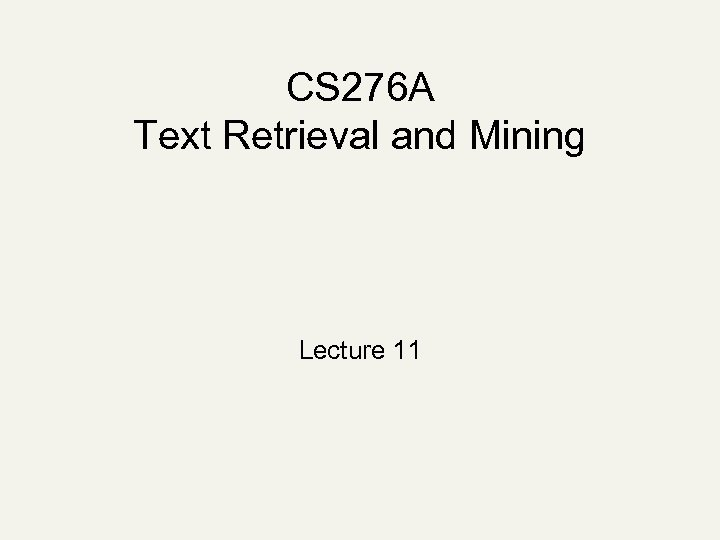CS 276 A Text Retrieval and Mining Lecture 11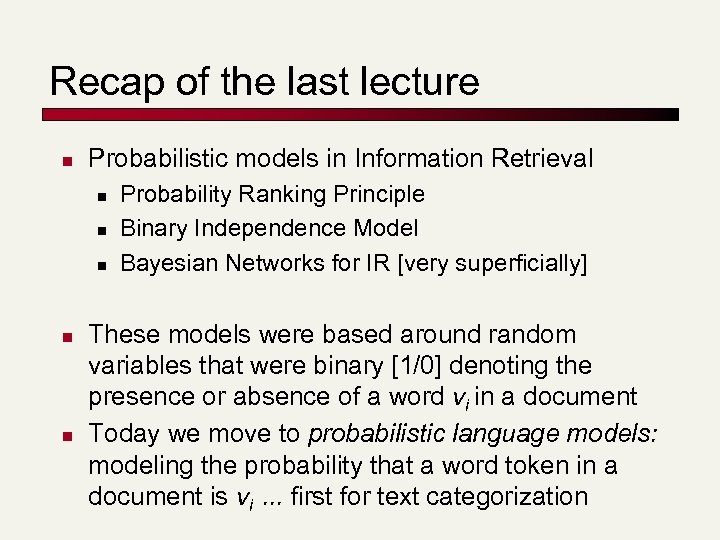Recap of the last lecture n Probabilistic models in Information Retrieval n n n Probability Ranking Principle Binary Independence Model Bayesian Networks for IR [very superficially] These models were based around random variables that were binary [1/0] denoting the presence or absence of a word vi in a document Today we move to probabilistic language models: modeling the probability that a word token in a document is vi. . . first for text categorization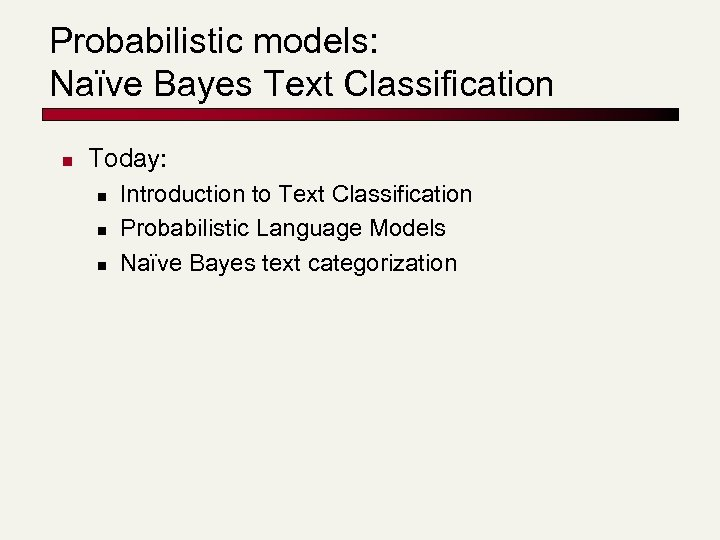Probabilistic models: Naïve Bayes Text Classification n Today: n n n Introduction to Text Classification Probabilistic Language Models Naïve Bayes text categorization

Subject: real estate is the only way." src="https://present5.com/presentation/e013eec037d54ce95171f51c862242e9/image-4.jpg" alt="Is this spam? From: "" Subject: real estate is the only way." /> Is this spam? From: "" Subject: real estate is the only way. . . gem oalvgkay Anyone can buy real estate with no money down Stop paying rent TODAY ! There is no need to spend hundreds or even thousands for similar courses I am 22 years old and I have already purchased 6 properties using the methods outlined in this truly INCREDIBLE ebook. Change your life NOW ! ========================= Click Below to order: http: //www. wholesaledaily. com/sales/nmd. htm =========================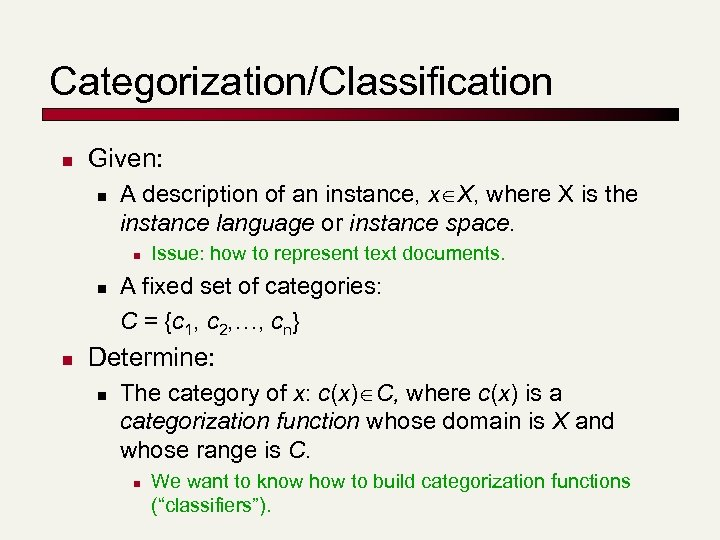Categorization/Classification n Given: n A description of an instance, x X, where X is the instance language or instance space. n n n Issue: how to represent text documents. A fixed set of categories: C = {c 1, c 2, …, cn} Determine: n The category of x: c(x) C, where c(x) is a categorization function whose domain is X and whose range is C. n We want to know how to build categorization functions (“classifiers”).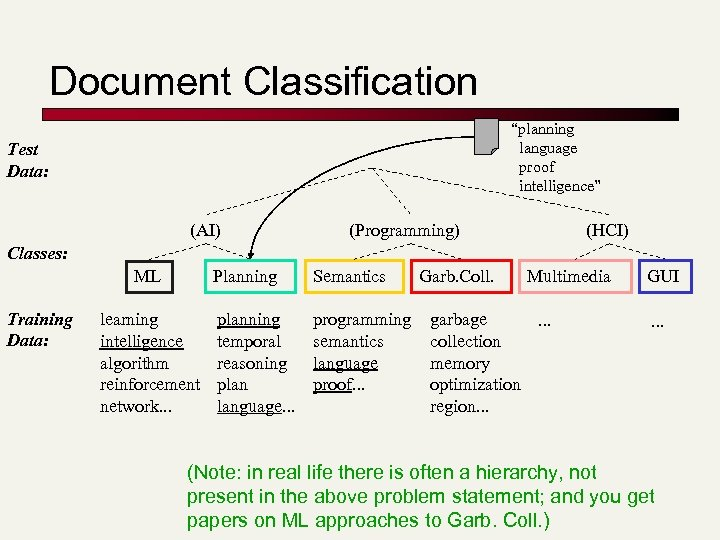Document Classification “planning language proof intelligence” Test Data: (AI) (Programming) (HCI) Classes: Planning ML Training Data: learning intelligence algorithm reinforcement network. . . Semantics planning temporal reasoning plan language. . . programming semantics language proof. . . Garb. Coll. Multimedia garbage. . . collection memory optimization region. . . GUI. . . (Note: in real life there is often a hierarchy, not present in the above problem statement; and you get papers on ML approaches to Garb. Coll. )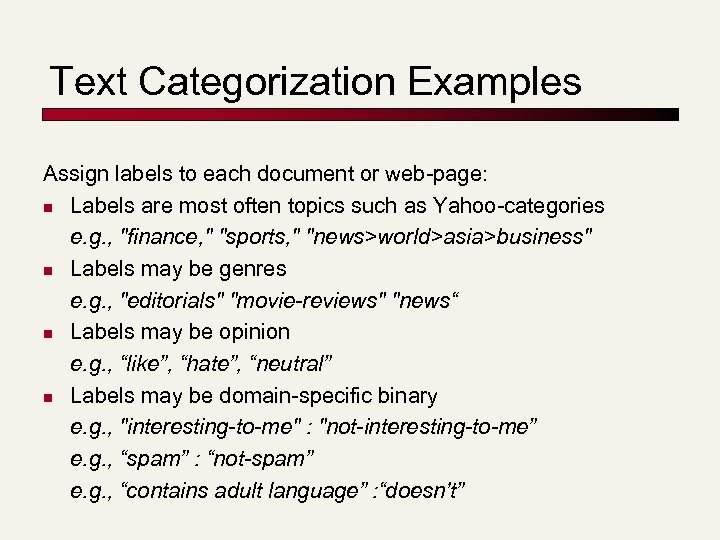Text Categorization Examples Assign labels to each document or web-page: n Labels are most often topics such as Yahoo-categories e. g. , "finance, " "sports, " "news>world>asia>business" n Labels may be genres e. g. , "editorials" "movie-reviews" "news“ n Labels may be opinion e. g. , “like”, “hate”, “neutral” n Labels may be domain-specific binary e. g. , "interesting-to-me" : "not-interesting-to-me” e. g. , “spam” : “not-spam” e. g. , “contains adult language” : “doesn’t”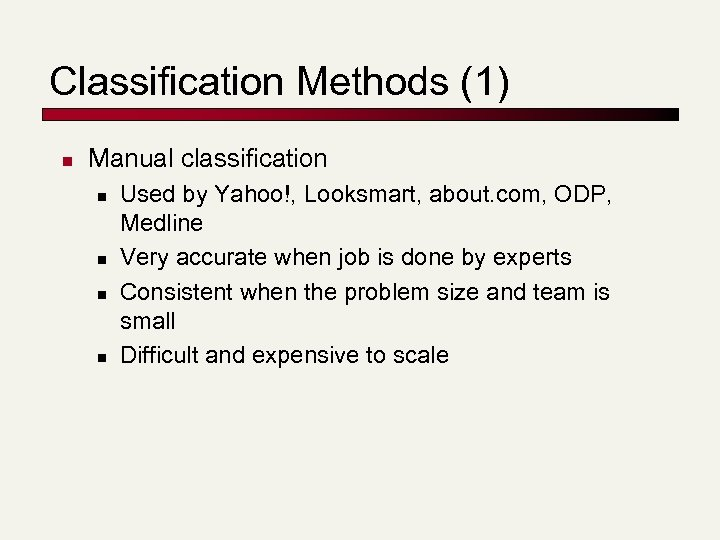Classification Methods (1) n Manual classification n n Used by Yahoo!, Looksmart, about. com, ODP, Medline Very accurate when job is done by experts Consistent when the problem size and team is small Difficult and expensive to scale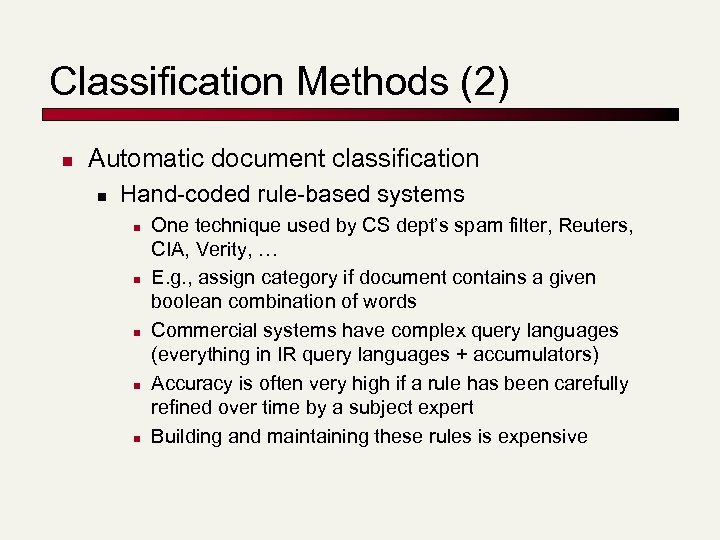Classification Methods (2) n Automatic document classification n Hand-coded rule-based systems n n n One technique used by CS dept’s spam filter, Reuters, CIA, Verity, … E. g. , assign category if document contains a given boolean combination of words Commercial systems have complex query languages (everything in IR query languages + accumulators) Accuracy is often very high if a rule has been carefully refined over time by a subject expert Building and maintaining these rules is expensive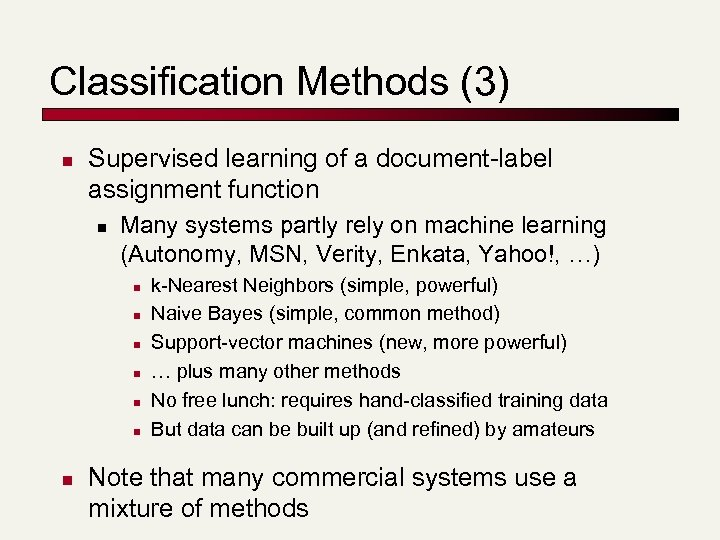Classification Methods (3) n Supervised learning of a document-label assignment function n Many systems partly rely on machine learning (Autonomy, MSN, Verity, Enkata, Yahoo!, …) n n n n k-Nearest Neighbors (simple, powerful) Naive Bayes (simple, common method) Support-vector machines (new, more powerful) … plus many other methods No free lunch: requires hand-classified training data But data can be built up (and refined) by amateurs Note that many commercial systems use a mixture of methods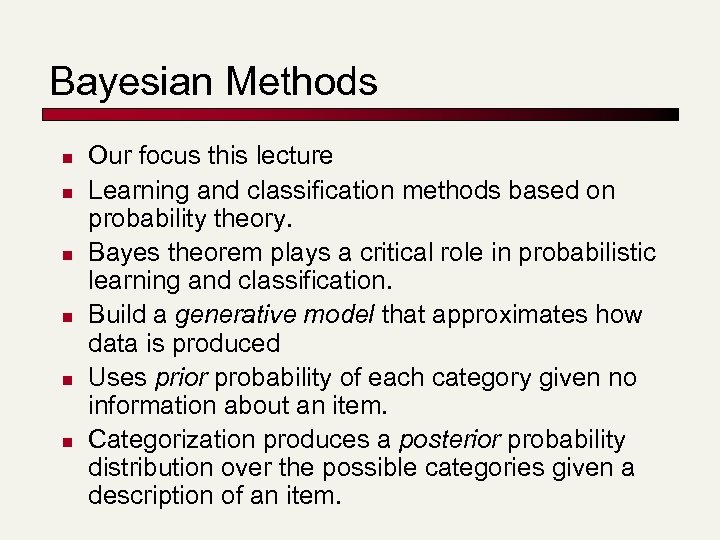Bayesian Methods n n n Our focus this lecture Learning and classification methods based on probability theory. Bayes theorem plays a critical role in probabilistic learning and classification. Build a generative model that approximates how data is produced Uses prior probability of each category given no information about an item. Categorization produces a posterior probability distribution over the possible categories given a description of an item.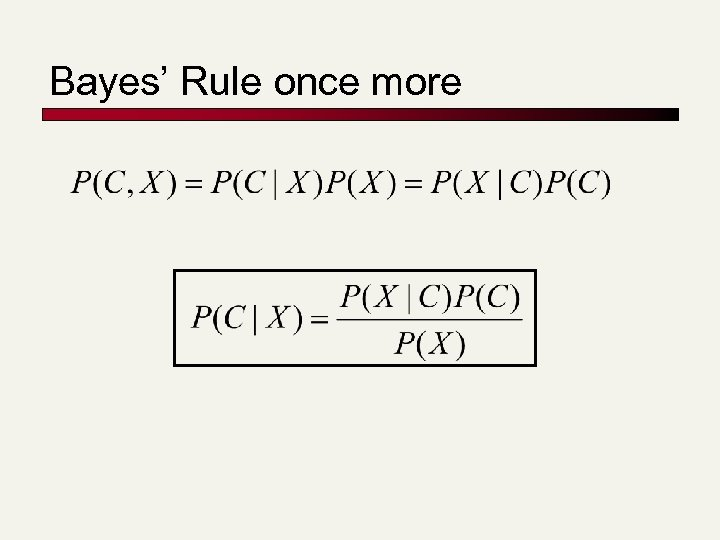Bayes’ Rule once more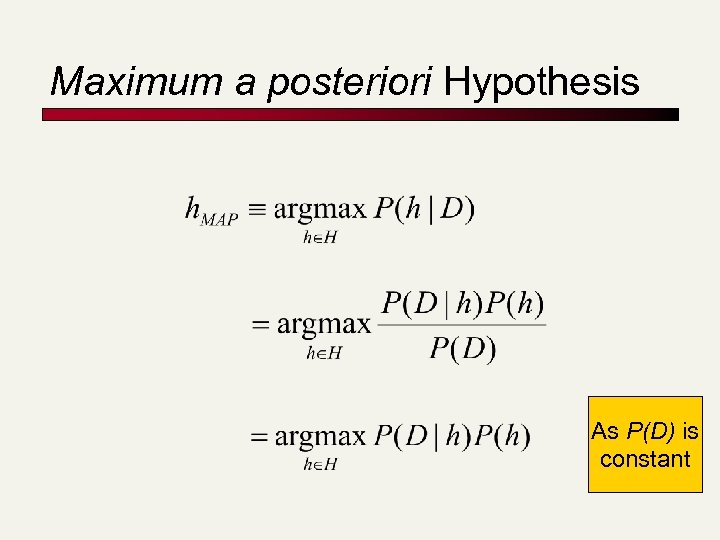Maximum a posteriori Hypothesis As P(D) is constant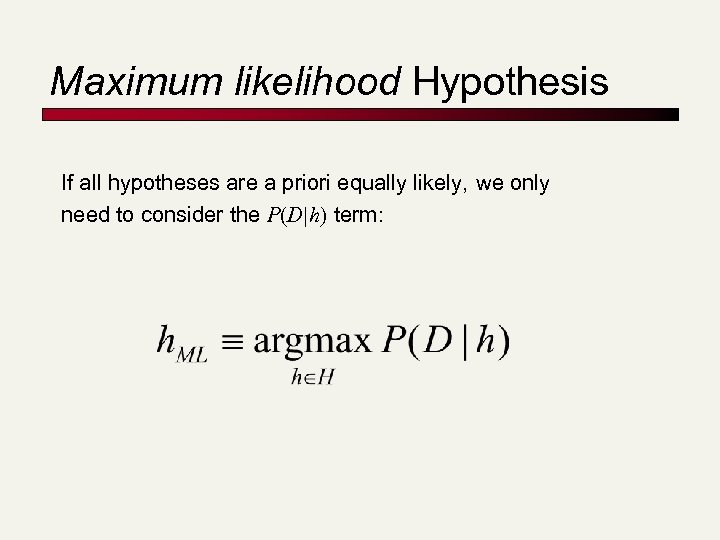Maximum likelihood Hypothesis If all hypotheses are a priori equally likely, we only need to consider the P(D|h) term: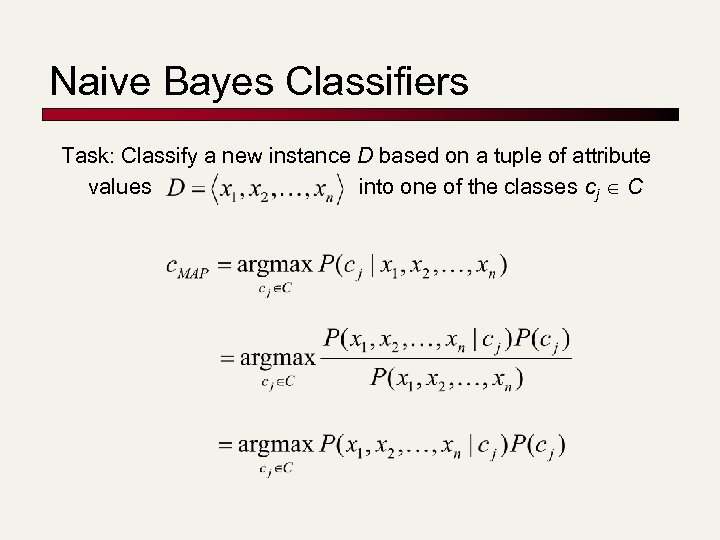Naive Bayes Classifiers Task: Classify a new instance D based on a tuple of attribute values into one of the classes cj C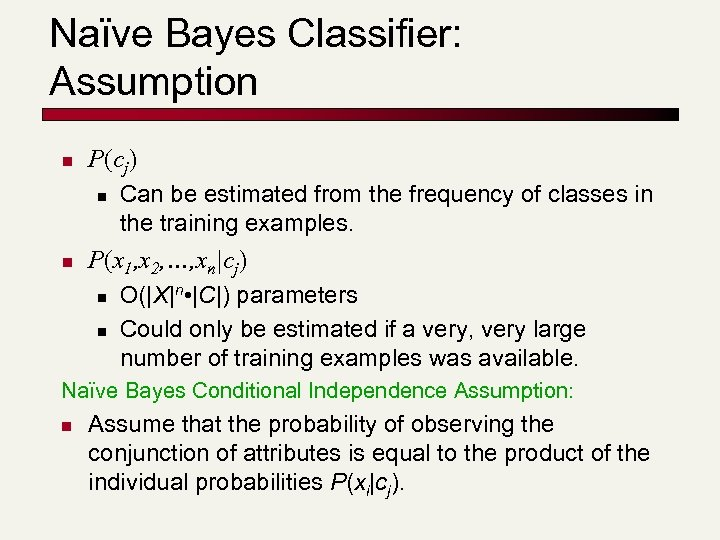Naïve Bayes Classifier: Assumption n P(cj) n n Can be estimated from the frequency of classes in the training examples. P(x 1, x 2, …, xn|cj) n n O(|X|n • |C|) parameters Could only be estimated if a very, very large number of training examples was available. Naïve Bayes Conditional Independence Assumption: n Assume that the probability of observing the conjunction of attributes is equal to the product of the individual probabilities P(xi|cj).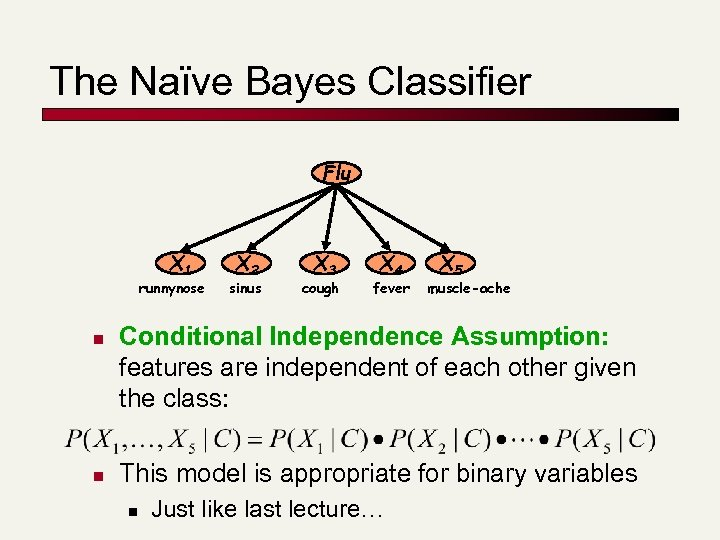The Naïve Bayes Classifier Flu X 1 runnynose n n X 2 sinus X 3 cough X 4 fever X 5 muscle-ache Conditional Independence Assumption: features are independent of each other given the class: This model is appropriate for binary variables n Just like last lecture…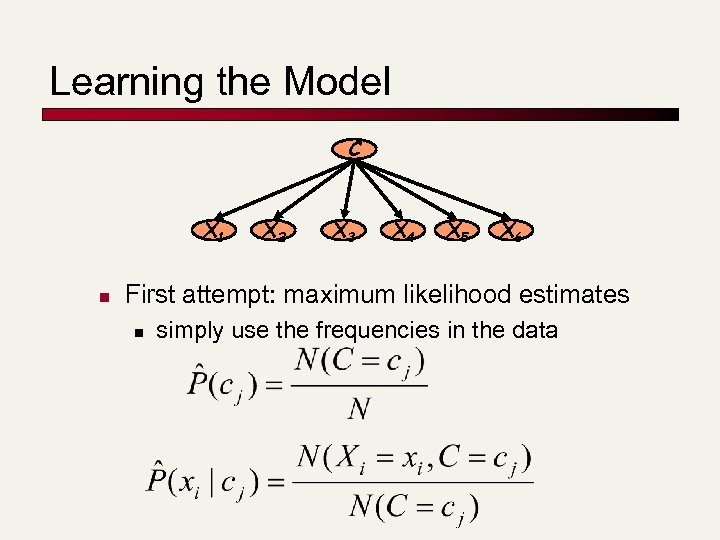Learning the Model C X 1 n X 2 X 3 X 4 X 5 X 6 First attempt: maximum likelihood estimates n simply use the frequencies in the data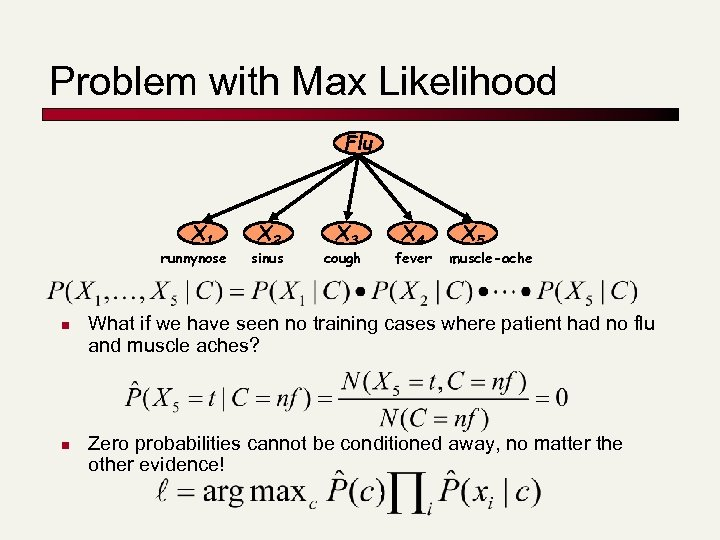Problem with Max Likelihood Flu X 1 runnynose n n X 2 sinus X 3 cough X 4 fever X 5 muscle-ache What if we have seen no training cases where patient had no flu and muscle aches? Zero probabilities cannot be conditioned away, no matter the other evidence!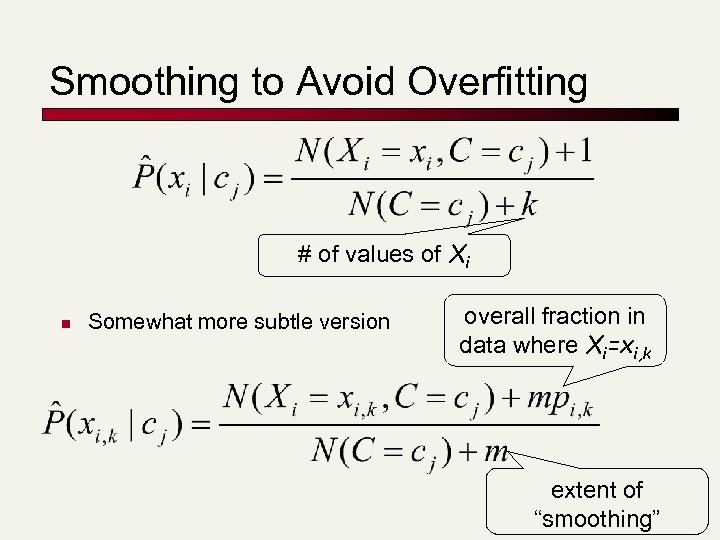Smoothing to Avoid Overfitting # of values of Xi n Somewhat more subtle version overall fraction in data where Xi=xi, k extent of “smoothing”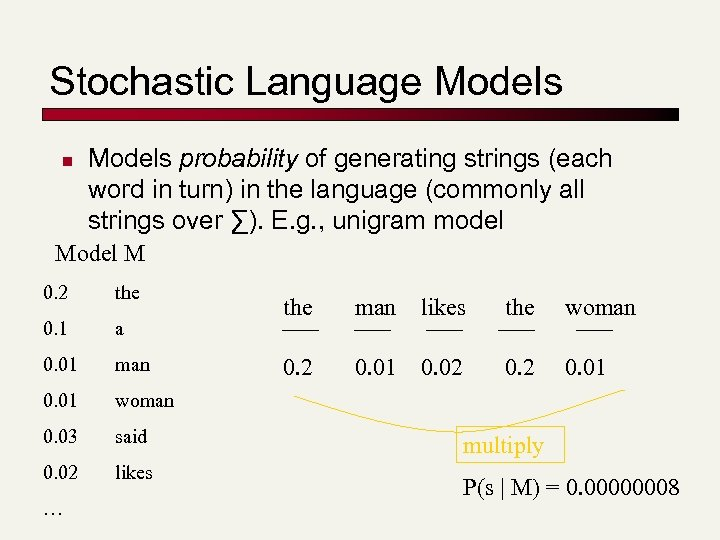Stochastic Language Models n Models probability of generating strings (each word in turn) in the language (commonly all strings over ∑). E. g. , unigram model M 0. 2 the 0. 1 a 0. 01 man 0. 01 woman 0. 03 said 0. 02 likes … the man likes the woman 0. 2 0. 01 0. 02 0. 01 multiply P(s | M) = 0. 00000008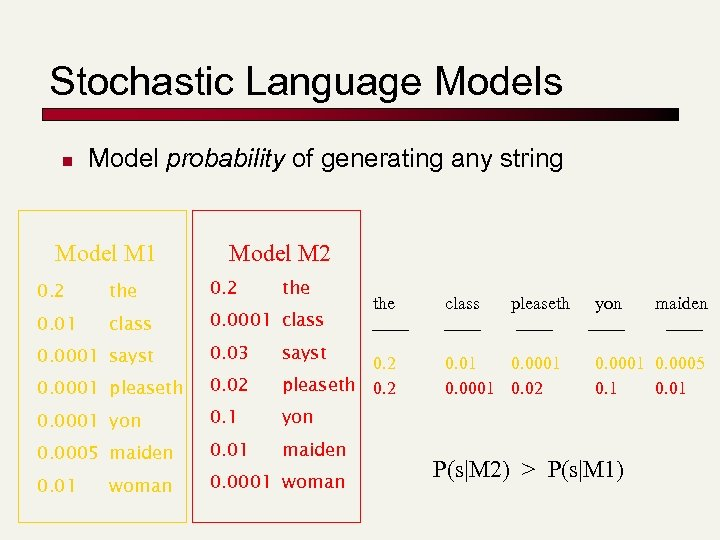Stochastic Language Models n Model probability of generating any string Model M 1 Model M 2 0. 2 the 0. 01 class 0. 0001 sayst 0. 03 0. 0001 pleaseth 0. 02 0. 2 pleaseth 0. 2 0. 0001 yon 0. 1 0. 0005 maiden 0. 01 0. 0001 woman class pleaseth yon woman sayst the 0. 01 0. 0001 0. 02 maiden 0. 0001 0. 0005 0. 1 0. 01 P(s|M 2) > P(s|M 1)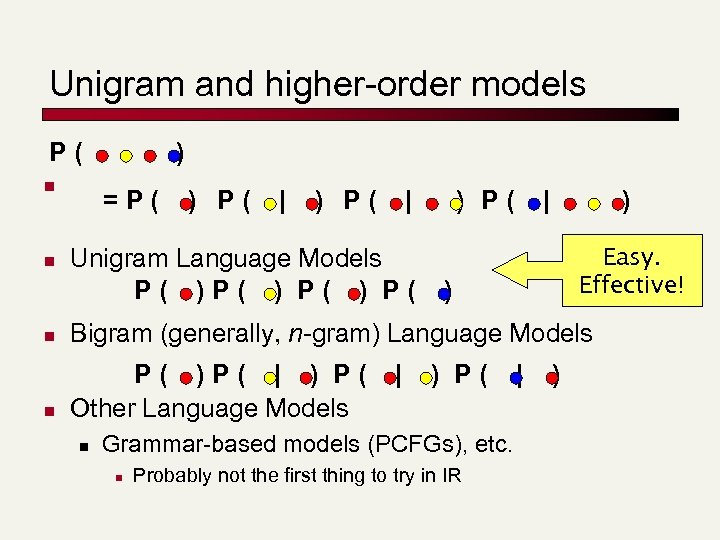Unigram and higher-order models P( n n ) =P( ) P( | Unigram Language Models P( ) P( | ) Easy. Effective! ) n Bigram (generally, n-gram) Language Models n P( )P( | ) P( Other Language Models n | ) P( Grammar-based models (PCFGs), etc. n Probably not the first thing to try in IR | )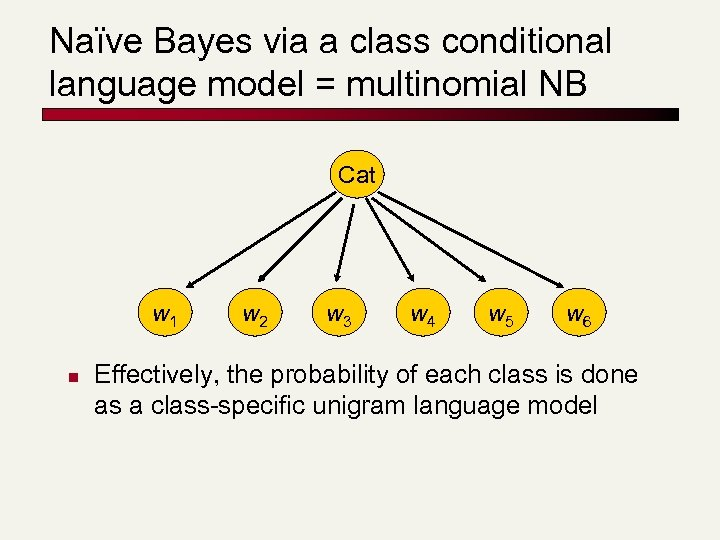Naïve Bayes via a class conditional language model = multinomial NB Cat w 1 n w 2 w 3 w 4 w 5 w 6 Effectively, the probability of each class is done as a class-specific unigram language model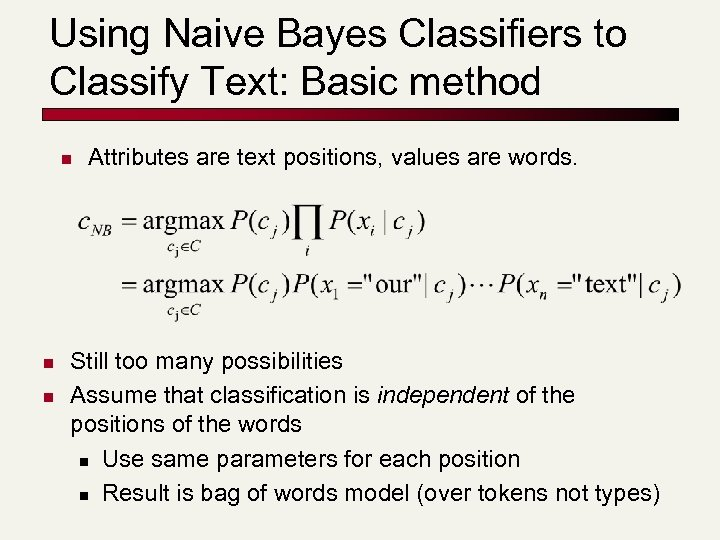Using Naive Bayes Classifiers to Classify Text: Basic method n n n Attributes are text positions, values are words. Still too many possibilities Assume that classification is independent of the positions of the words n Use same parameters for each position n Result is bag of words model (over tokens not types)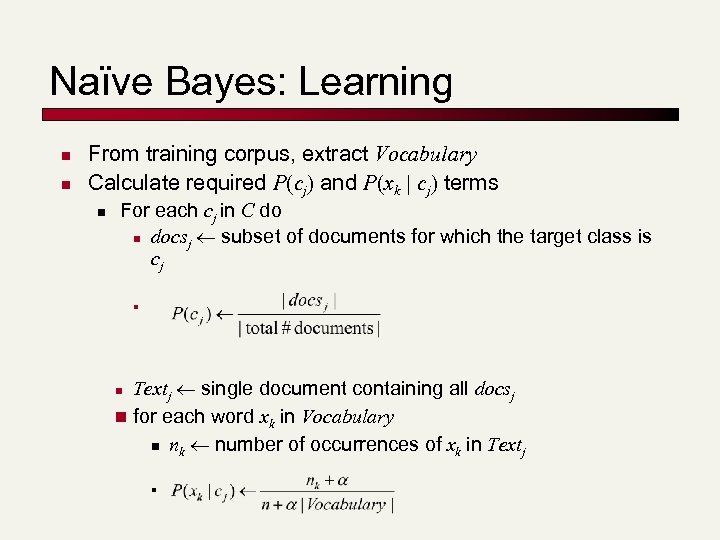Naïve Bayes: Learning n n From training corpus, extract Vocabulary Calculate required P(cj) and P(xk | cj) terms n For each cj in C do n docsj subset of documents for which the target class is cj n Textj single document containing all docsj n for each word xk in Vocabulary n nk number of occurrences of xk in Textj n n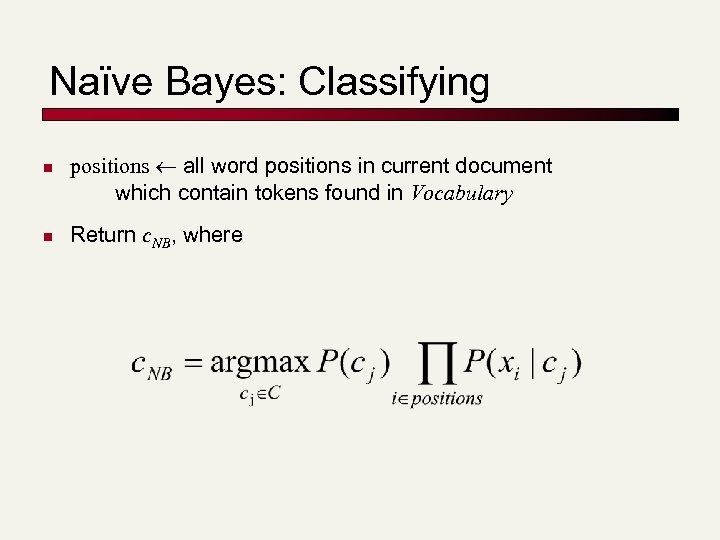Naïve Bayes: Classifying n n positions all word positions in current document which contain tokens found in Vocabulary Return c. NB, where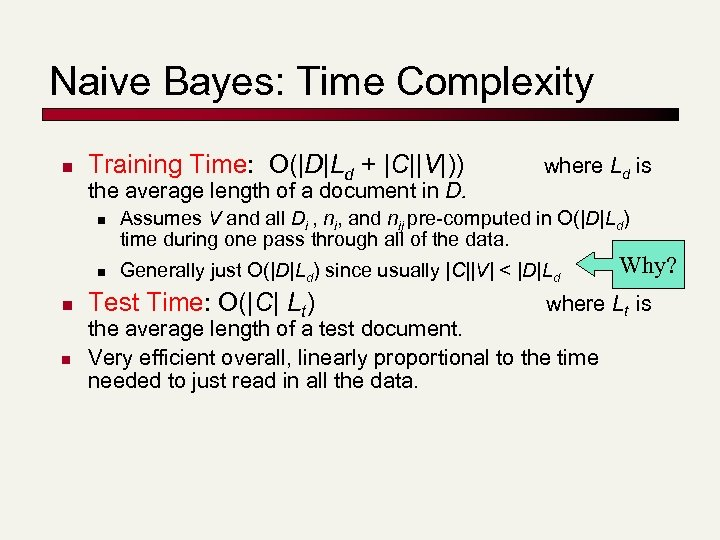Naive Bayes: Time Complexity n Training Time: O(|D|Ld + |C||V|)) the average length of a document in D. n n where Ld is Assumes V and all Di , ni, and nij pre-computed in O(|D|Ld) time during one pass through all of the data. Generally just O(|D|Ld) since usually |C||V| < |D|Ld Test Time: O(|C| Lt) Why? where Lt is the average length of a test document. Very efficient overall, linearly proportional to the time needed to just read in all the data.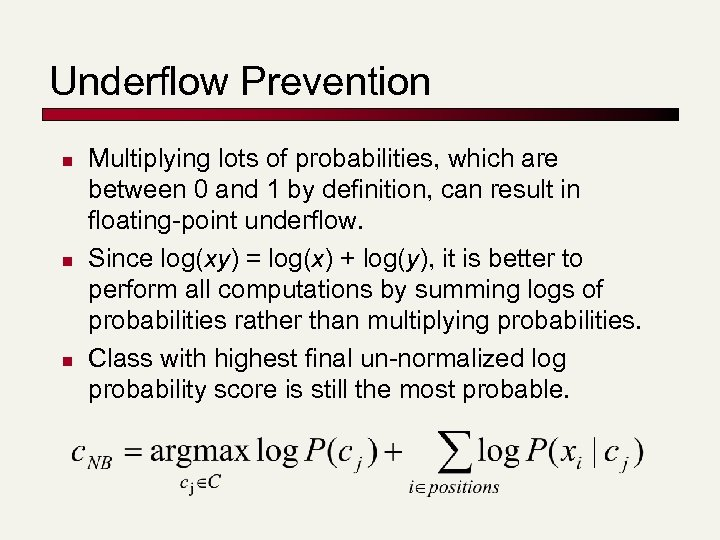Underflow Prevention n Multiplying lots of probabilities, which are between 0 and 1 by definition, can result in floating-point underflow. Since log(xy) = log(x) + log(y), it is better to perform all computations by summing logs of probabilities rather than multiplying probabilities. Class with highest final un-normalized log probability score is still the most probable.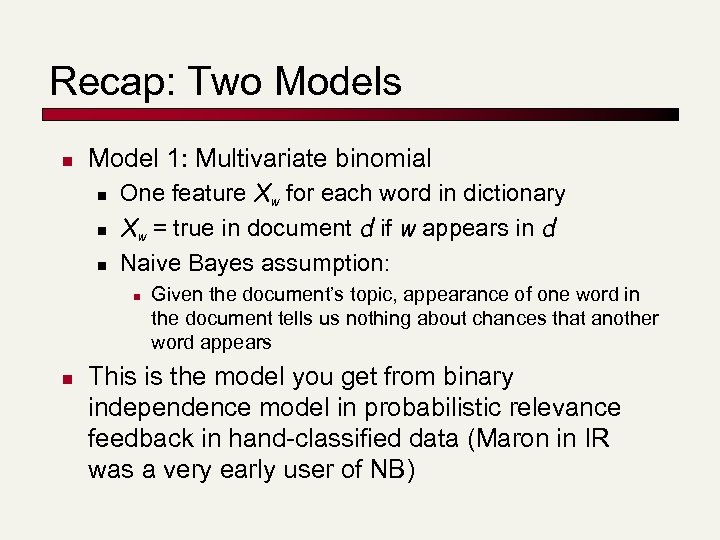Recap: Two Models n Model 1: Multivariate binomial n n n One feature Xw for each word in dictionary Xw = true in document d if w appears in d Naive Bayes assumption: n n Given the document’s topic, appearance of one word in the document tells us nothing about chances that another word appears This is the model you get from binary independence model in probabilistic relevance feedback in hand-classified data (Maron in IR was a very early user of NB)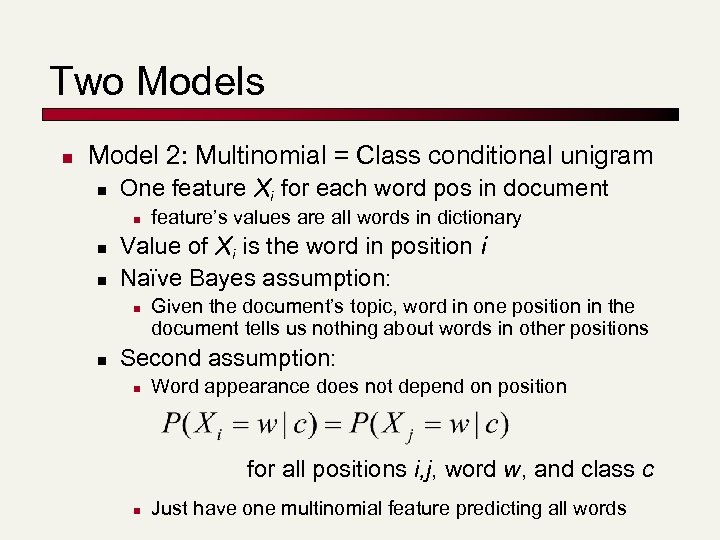Two Models n Model 2: Multinomial = Class conditional unigram n One feature Xi for each word pos in document n n n Value of Xi is the word in position i Naïve Bayes assumption: n n feature’s values are all words in dictionary Given the document’s topic, word in one position in the document tells us nothing about words in other positions Second assumption: n Word appearance does not depend on position for all positions i, j, word w, and class c n Just have one multinomial feature predicting all words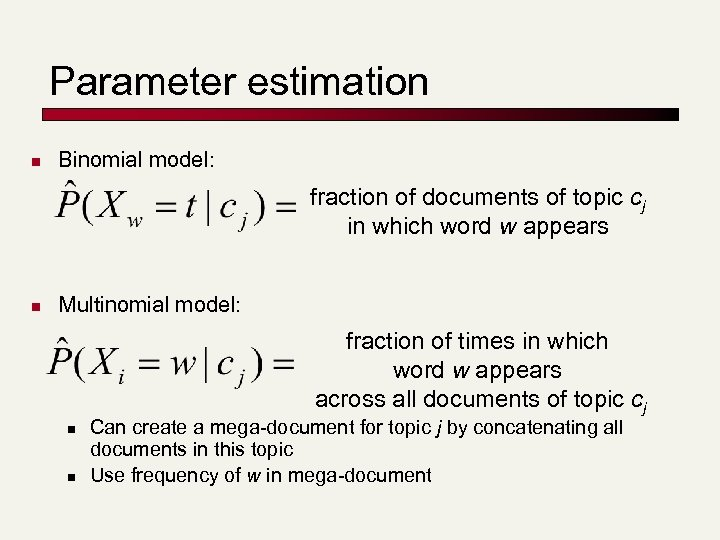Parameter estimation n Binomial model: fraction of documents of topic cj in which word w appears n Multinomial model: fraction of times in which word w appears across all documents of topic cj n n Can create a mega-document for topic j by concatenating all documents in this topic Use frequency of w in mega-document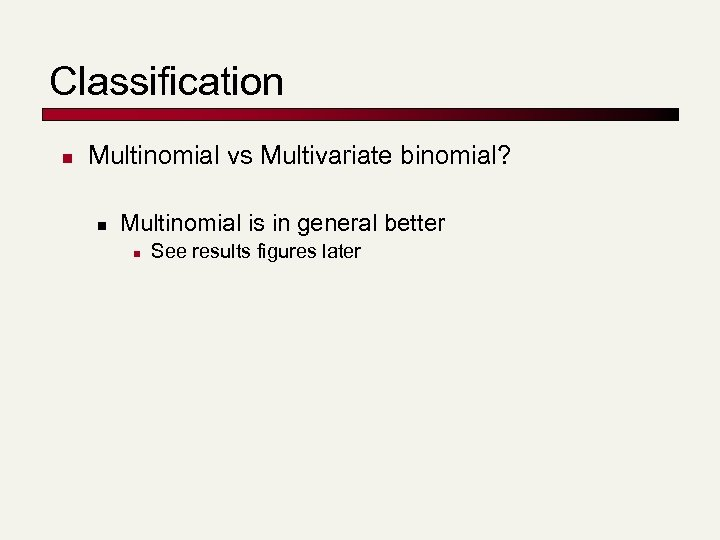Classification n Multinomial vs Multivariate binomial? n Multinomial is in general better n See results figures later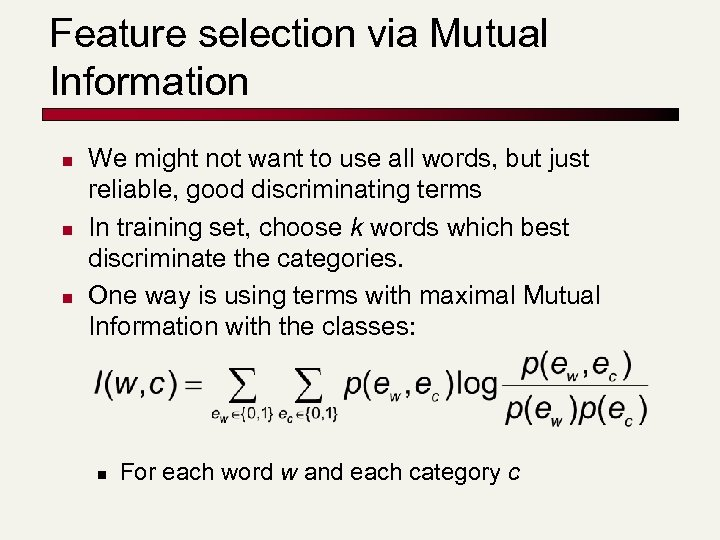Feature selection via Mutual Information n We might not want to use all words, but just reliable, good discriminating terms In training set, choose k words which best discriminate the categories. One way is using terms with maximal Mutual Information with the classes: n For each word w and each category c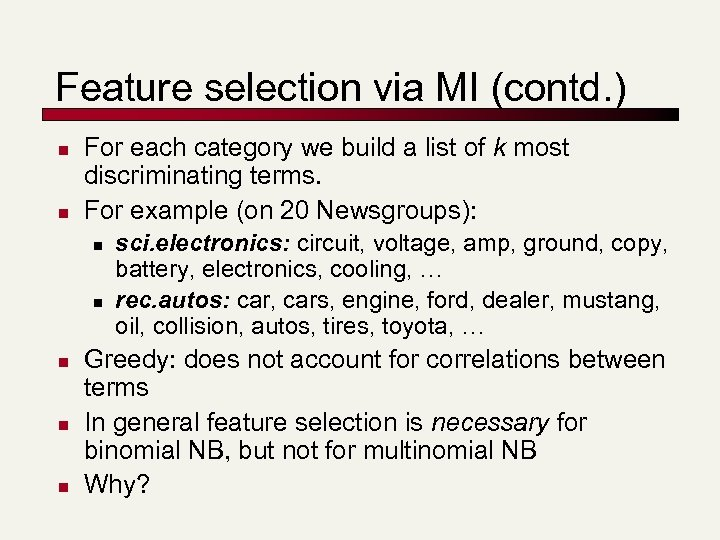Feature selection via MI (contd. ) n n For each category we build a list of k most discriminating terms. For example (on 20 Newsgroups): n n n sci. electronics: circuit, voltage, amp, ground, copy, battery, electronics, cooling, … rec. autos: car, cars, engine, ford, dealer, mustang, oil, collision, autos, tires, toyota, … Greedy: does not account for correlations between terms In general feature selection is necessary for binomial NB, but not for multinomial NB Why?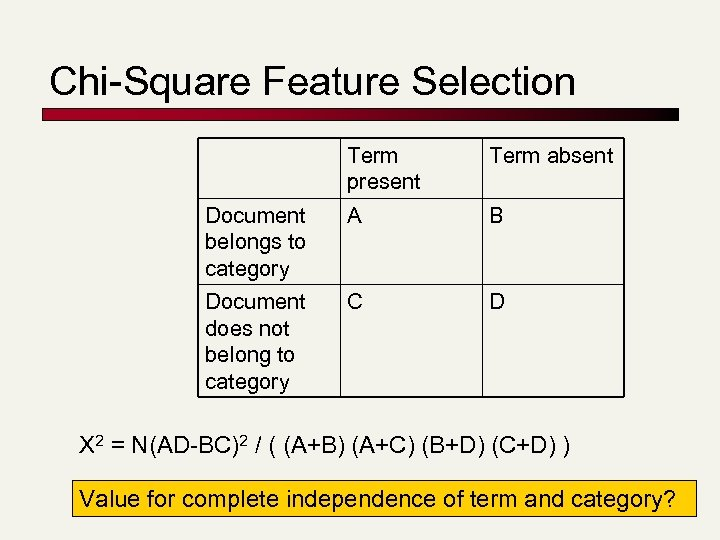Chi-Square Feature Selection Term present Term absent Document belongs to category A B Document does not belong to category C D X 2 = N(AD-BC)2 / ( (A+B) (A+C) (B+D) (C+D) ) Value for complete independence of term and category?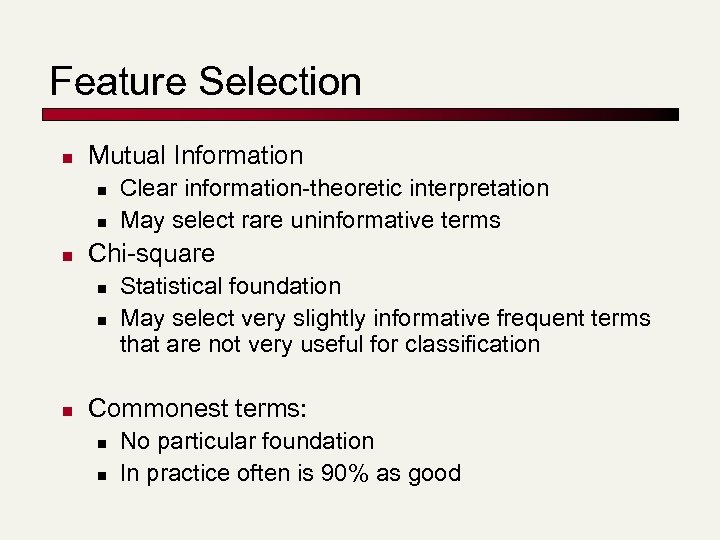Feature Selection n Mutual Information n Chi-square n n n Clear information-theoretic interpretation May select rare uninformative terms Statistical foundation May select very slightly informative frequent terms that are not very useful for classification Commonest terms: n n No particular foundation In practice often is 90% as good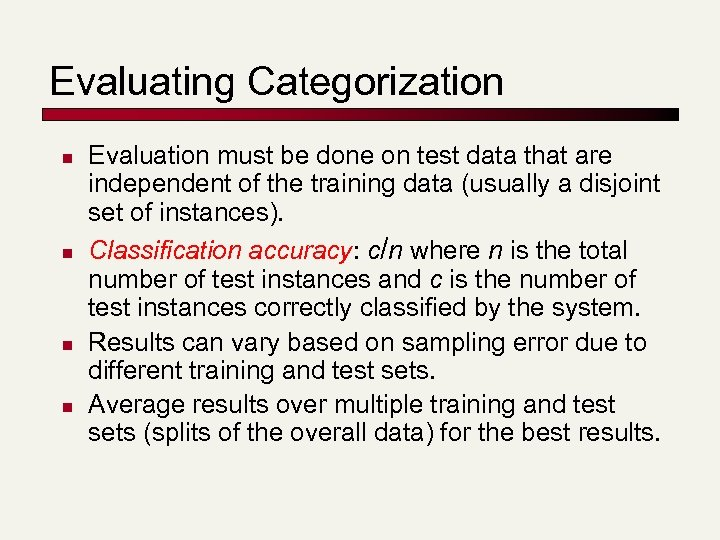Evaluating Categorization n n Evaluation must be done on test data that are independent of the training data (usually a disjoint set of instances). Classification accuracy: c/n where n is the total number of test instances and c is the number of test instances correctly classified by the system. Results can vary based on sampling error due to different training and test sets. Average results over multiple training and test sets (splits of the overall data) for the best results.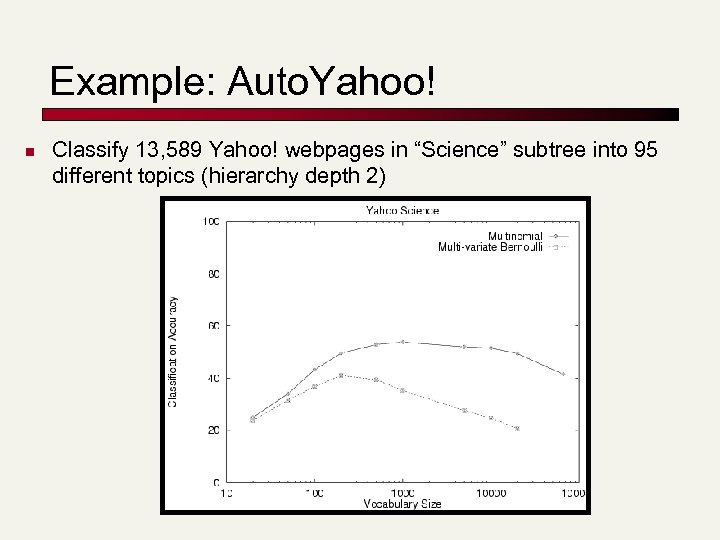Example: Auto. Yahoo! n Classify 13, 589 Yahoo! webpages in “Science” subtree into 95 different topics (hierarchy depth 2)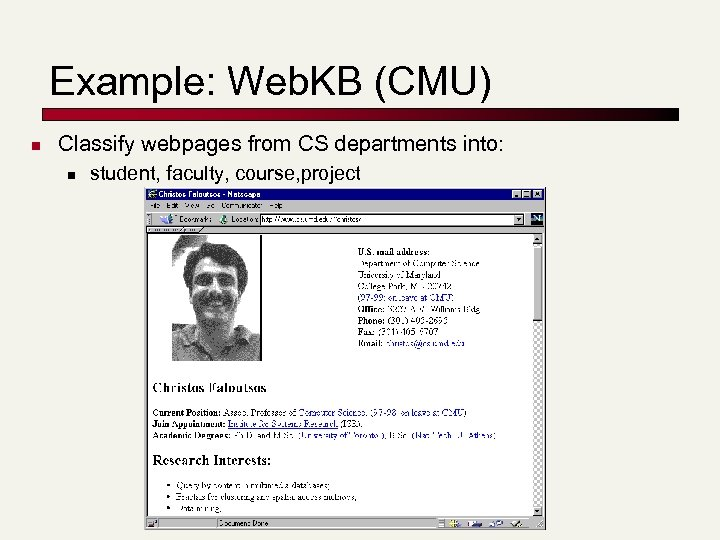Example: Web. KB (CMU) n Classify webpages from CS departments into: n student, faculty, course, project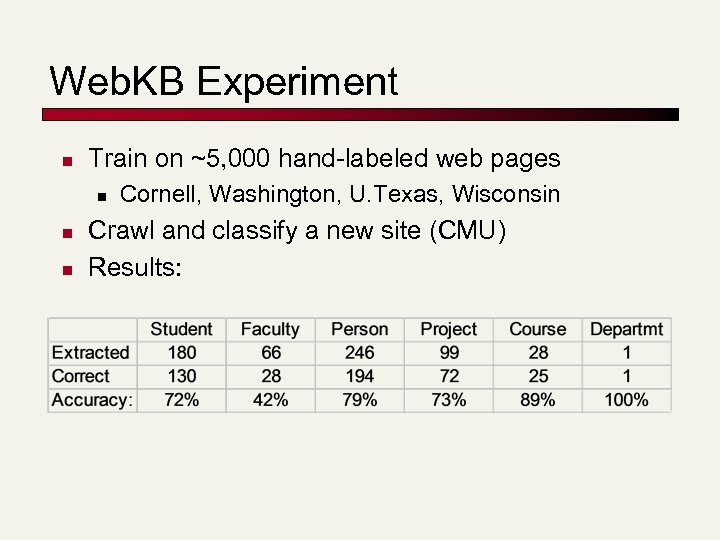Web. KB Experiment n Train on ~5, 000 hand-labeled web pages n n n Cornell, Washington, U. Texas, Wisconsin Crawl and classify a new site (CMU) Results: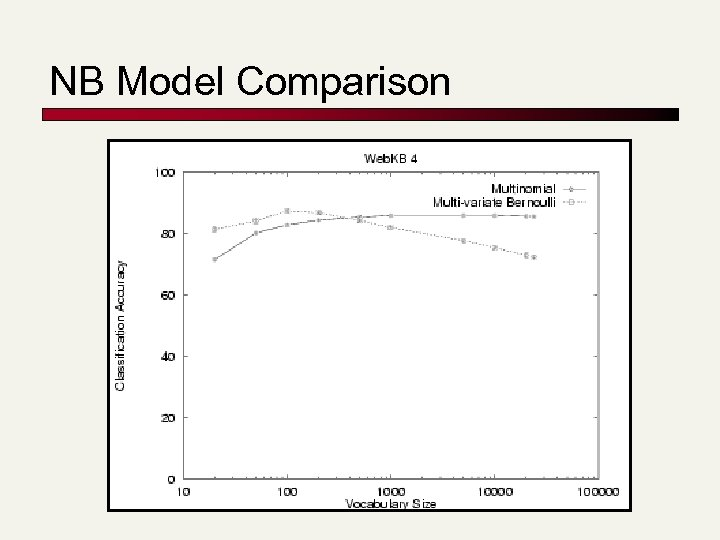NB Model Comparison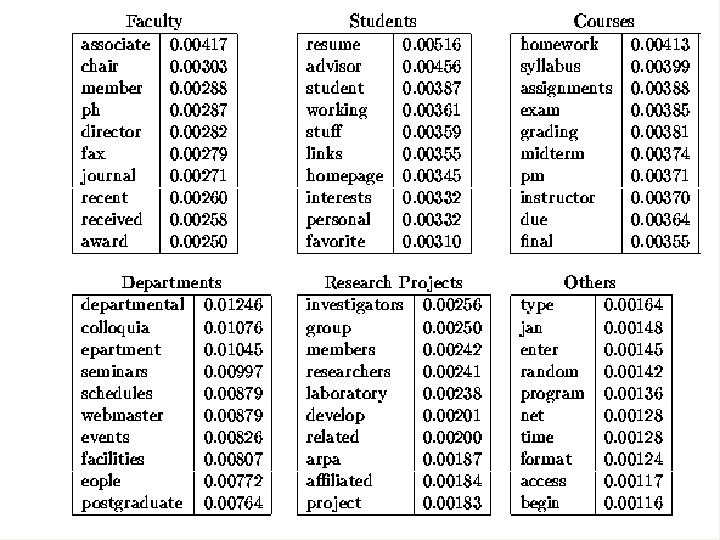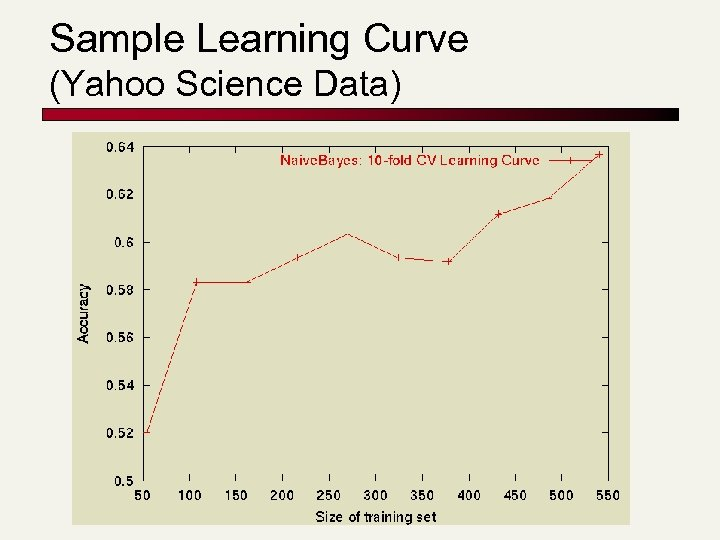Sample Learning Curve (Yahoo Science Data)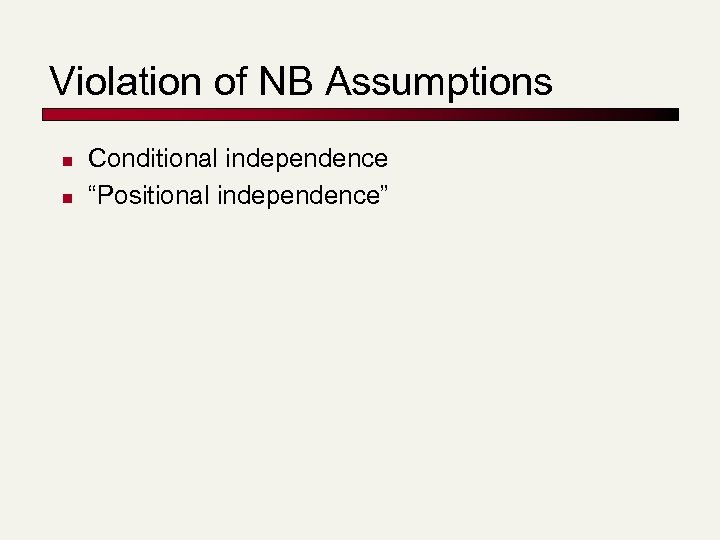Violation of NB Assumptions n n Conditional independence “Positional independence”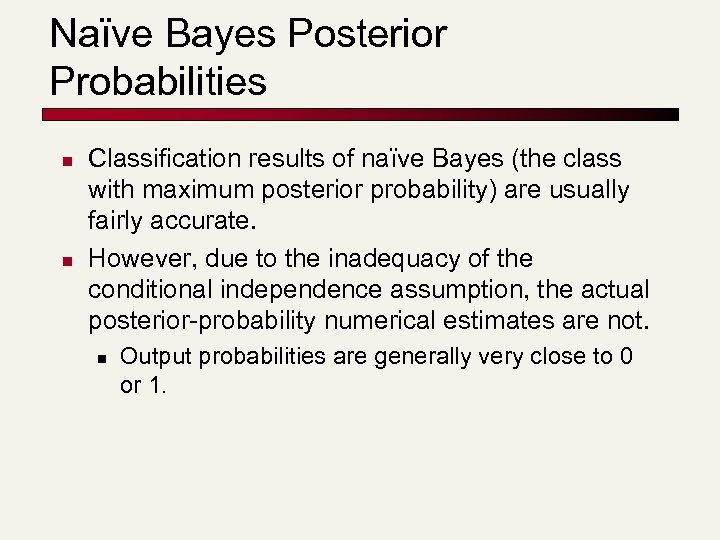Naïve Bayes Posterior Probabilities n n Classification results of naïve Bayes (the class with maximum posterior probability) are usually fairly accurate. However, due to the inadequacy of the conditional independence assumption, the actual posterior-probability numerical estimates are not. n Output probabilities are generally very close to 0 or 1.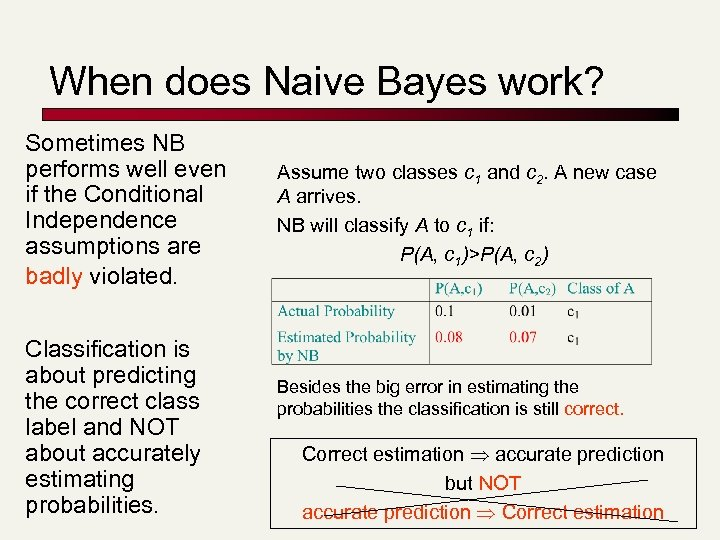When does Naive Bayes work? Sometimes NB performs well even if the Conditional Independence assumptions are badly violated. Classification is about predicting the correct class label and NOT about accurately estimating probabilities. Assume two classes c 1 and c 2. A new case A arrives. NB will classify A to c 1 if: P(A, c 1)>P(A, c 2) Besides the big error in estimating the probabilities the classification is still correct. Correct estimation accurate prediction but NOT accurate prediction Correct estimation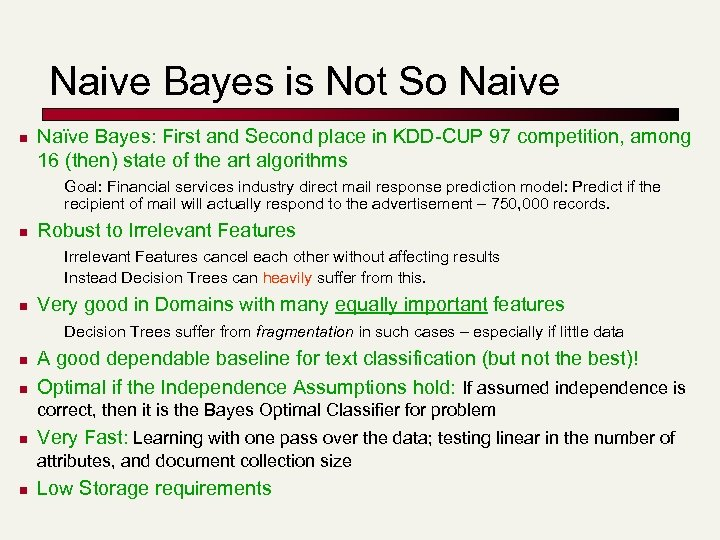Naive Bayes is Not So Naive n Naïve Bayes: First and Second place in KDD-CUP 97 competition, among 16 (then) state of the art algorithms Goal: Financial services industry direct mail response prediction model: Predict if the recipient of mail will actually respond to the advertisement – 750, 000 records. n Robust to Irrelevant Features cancel each other without affecting results Instead Decision Trees can heavily suffer from this. n Very good in Domains with many equally important features Decision Trees suffer from fragmentation in such cases – especially if little data n n A good dependable baseline for text classification (but not the best)! Optimal if the Independence Assumptions hold: If assumed independence is correct, then it is the Bayes Optimal Classifier for problem n Very Fast: Learning with one pass over the data; testing linear in the number of attributes, and document collection size n Low Storage requirements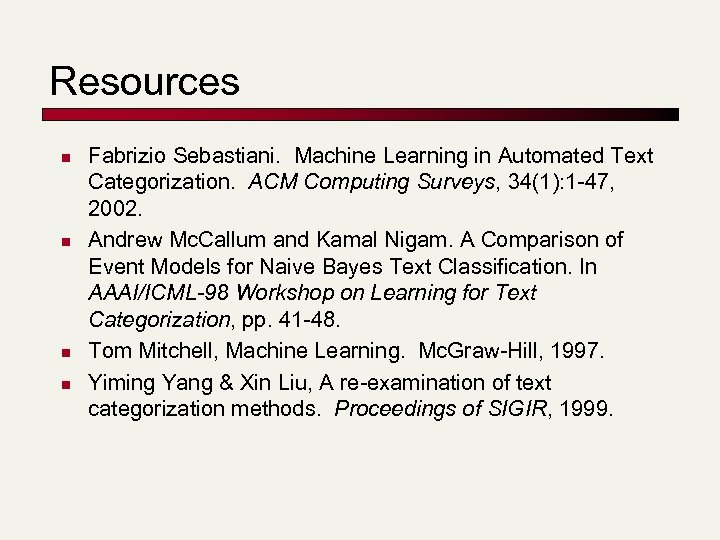Resources n n Fabrizio Sebastiani. Machine Learning in Automated Text Categorization. ACM Computing Surveys, 34(1): 1 -47, 2002. Andrew Mc. Callum and Kamal Nigam. A Comparison of Event Models for Naive Bayes Text Classification. In AAAI/ICML-98 Workshop on Learning for Text Categorization, pp. 41 -48. Tom Mitchell, Machine Learning. Mc. Graw-Hill, 1997. Yiming Yang & Xin Liu, A re-examination of text categorization methods. Proceedings of SIGIR, 1999.